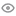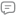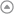New update is available. Click here to update.Netaji Subhas University Of Technology 2024
My StatsEXP gained2520Level
5 (Champion)
Community statsDiscussions
00
Know more41
Total problems solved
15
Easy
23
Moderate
3
Hard
0
NinjaCurrent streak:

0 days

Longest streak:

1 day

Less

More

Discussions
my solution
Interview problems
``````/*
Time Complexity: O(N)
Space Complexity: O(N)

Where 'N' is the length of the string.
*/

// Make a new node taking operator top as root and right and left child from tree stack.
void makeNode(stack<char> &op, stack<BinaryTreeNode<char> *> &tree)
{
BinaryTreeNode<char> *root = new BinaryTreeNode<char>(op.top());

op.pop();
root->right = tree.top();
tree.pop();
root->left = tree.top();
tree.pop();
tree.push(root);
}

// Check whether a character is between 0 and 9.
bool isDigit(char c)
{
if ('0' <= c && c <= '9')
{
return 1;
}
return 0;
}

BinaryTreeNode<char> *binaryExpressionTree(string s)
{
map<char, int> priority;

priority['('] = 1;
priority['-'] = 2;
priority['+'] = 2;
priority['*'] = 3;
priority['/'] = 3;

stack<BinaryTreeNode<char> *> tree;
stack<char> operators;
for (int i = 0; i < s.length(); i++)
{

if (s[i] == '(')
{
operators.push('(');
}
else if (isDigit(s[i]))
{
BinaryTreeNode<char> *Node = new BinaryTreeNode<char>(s[i]);
tree.push(Node);
}
else if (s[i] == ')')
{
while (operators.top() != '(')
{
makeNode(operators, tree);
}

operators.pop();
}
else
{
while (!operators.empty() && priority[operators.top()] >= priority[s[i]])
{
makeNode(operators, tree);
}

operators.push(s[i]);
}
}

while (tree.size() > 1)
{
makeNode(operators, tree);
}

return tree.top();
}``````
Mohit Ahlawat
Published On 21-Aug-202322 views0 replies